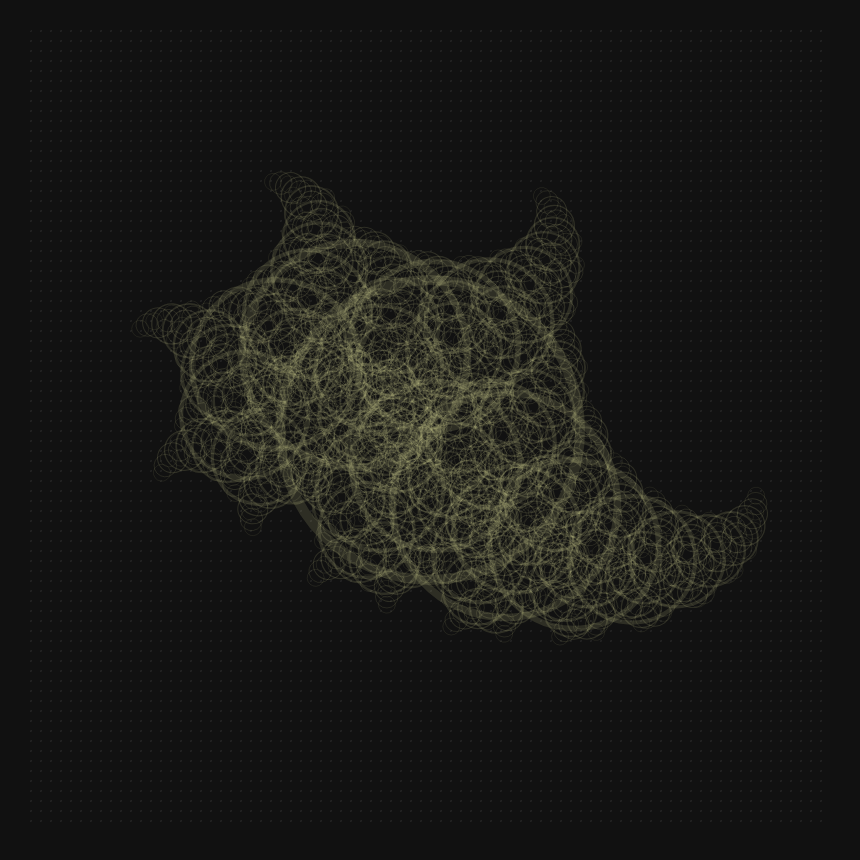## Ronin experiment 12 - traces of a restless ghost

I created a fractal using a recursive function and animated the rotation anglesthis is the recursive function I used to create the animation

``````(defn rec (v) (
(def s 150)
(if (gt v 0) (
(stroke
(circle 0 0 s s)
"#FFFAAF20" 10)

(pushTransform)
(move (div s -2) (div s -2))
(scale 0.75)
(rotate (sin (time 0.00074)))
(rec (sub v 1))
(popTransform)
(pushTransform)
(move (div s 2) (div s 2))
(scale 0.75)
(rotate (sin (time 0.00053)))

(rec (sub v 1))
(popTransform)

))
))
(resize 800 800)
(on "animate" '(
(resetTransform)
(clear)
(move 400 400)
(rec 11)
))
``````